### DIFFERENTIATION USING THE CHAIN RULE

The following problems require the use of the chain rule. The chain rule is a rule for differentiating compositions of functions. In the following discussion and solutions the derivative of a function h(x) will be denoted byor h'(x) . Most problems are average. A few are somewhat challenging. The chain rule states formally that.

However, we rarely use this formal approach when applying the chain rule to specific problems. Instead, we invoke an intuitive approach. For example, it is sometimes easier to think of the functions f and g as ``layers'' of a problem. Function f is the ``outer layer'' and function g is the ``inner layer.'' Thus, the chain rule tells us to first differentiate the outer layer, leaving the inner layer unchanged (the term f'( g(x) ) ) , then differentiate the inner layer (the term g'(x) ) . This process will become clearer as you do the problems. In most cases, final answers are given in the most simplified form.

• PROBLEM 1 : Differentiate.

• PROBLEM 2 : Differentiate.

• PROBLEM 3 : Differentiate.

• PROBLEM 4 : Differentiate.

• PROBLEM 5 : Differentiate.

• PROBLEM 6 : Differentiate.

• PROBLEM 7 : Differentiate.

• PROBLEM 8 : Differentiate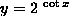.

• PROBLEM 9 : Differentiate.

• PROBLEM 10 : Differentiate.

• PROBLEM 11 : Differentiate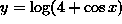.

### The following seven problems require more than one application of the chain rule.

• PROBLEM 12 : Differentiate.

• PROBLEM 13 : Differentiate.

• PROBLEM 14 : Differentiate.

• PROBLEM 15 : Differentiate.

• PROBLEM 16 : Differentiate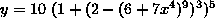.

• PROBLEM 17 : Differentiate.

• PROBLEM 18 : Differentiate.

### The following three problems require a more formal use of the chain rule.

• PROBLEM 19 : Assume that h(x) = f( g(x) ) , where both f and g are differentiable functions. If g(-1)=2, g'(-1)=3, and f'(2)=-4 , what is the value of h'(-1) ?

• PROBLEM 20 : Assume that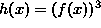, where f is a differentiable function. If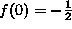and, determine an equation of the line tangent to the graph of h at x=0 .

• PROBLEM 21 : Determine a differentiable function y = f(x) which has the propertiesand.